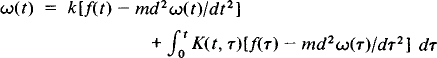Integro-Differential Equations

Integro-Differential Equations

equations containing both integrals and derivatives of an unknown function. An example is the equation obtained by the Italian mathematician V. Volterra in the problem of torsional oscillations:Sometimes integro-differential equations can be reduced to integral equations or differential equations. A solution to an integro-differential equation may be sought by the method of successive approximations.

References in periodicals archive ?
An improved method based on Haar wavelets for numerical solution of nonlinear integral and integro-differential equations of first and higher orders.
The applications they describe are numerical solutions of partial differential equations, integro-differential equations, image and signal processing, and time-series processing.
Staffans, A direct Lyapunov approach to Volterra integro-differential equations, SIAM J.
Goltser, "Stability of partial functional integro-differential equations," Journal of Dynamical and Control Systems, vol.
A quartic B-spline collocation method is proposed for numerical solution of partial integro-differential equations with a weakly singular kernel.
2010) "A series solution of the nonlinear Volterra and Fredholm integro-differential equations," Communications in Nonlinear Science and Numerical Simulation, vol.
Ghorbani, "He's homotopy perturbation method: an effective tool for solving nonlinear integral and integro-differential equations," Computers & Mathematics with Applications, vol.
Kaughten, The method of lines for parabolic partial integro-differential equations, J.
Work is was performed as part of the scientific program for basic research RK Ministry of Education "Solution new mathematical methods of nonlinear differential and integro-differential equations of fundamental and applied problems of mechanics of solid and deformable solids" (Contractfor research No.
Rao, Theory of Integro-differential Equations, Stability and Control: Theory, Methods and Applications 1, Gordon and Breach Publ.
Kanagarajan, Existence of solutions of fuzzy delay integro-differential equations with nonlocal condition, Journal of the Korea Society for Industrial and Applied Mathematics, 9 (2005) 65-74.
If an observation point is situated on an impedance body surface S then we arrive at a system of integro-differential equations

Site: Follow: Share:
Open / Close